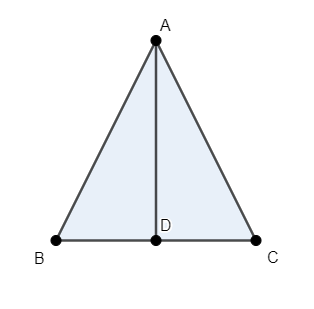Courses
Courses for Kids
Free study material
Free LIVE classes
MoreLIVE
Join Vedantu’s FREE Mastercalss

# $ABC$ is an equilateral triangle of side $a$. Find each of its altitudes.Verified
364.2k+ views
Hint: Draw perpendicular from one of the vertices to the side opposite to the vertex. Use the fact that the angles opposite to equal sides are equal to find the value of each angle of the equilateral triangle. Use trigonometric properties to relate the length of any one of the sides to the length of the altitude.

We have an equilateral triangle $\vartriangle ABC$ whose length of each of the sides is $a$. We have to evaluate the length of its altitudes. We must observe that all the altitudes of the equilateral triangle will be of the same length as each of the sides of the equilateral triangle are of equal length.

Consider the equilateral triangle $\vartriangle ABC$ whose length of each of the sides is $a$. Drop a perpendicular from any one of the vertices, say $A$ to the side opposite to the vertex, that is $BC$. Label the foot of the altitude on the side $BC$ as $D$, as shown in the figure.We know that the angles opposite to equal sides are equal.

As all the sides of an equilateral triangle are equal, the angles opposite to them are equal.

Thus, all the angles of an equilateral triangle are equal. Let’s assume this angle to be $\theta$.

As the sum of all the angles of a triangle is ${{180}^{\circ }}$, we have $3\theta ={{180}^{\circ }}$.
$\Rightarrow \theta ={{60}^{\circ }}$

Thus, the measure of each of the angles of an equilateral triangle is ${{60}^{\circ }}$.

To find the length of altitude $AD$, we will use trigonometric equations.
We will consider the triangle $\vartriangle ABD$. We know that measure of angle $\angle ABD={{60}^{\circ }}$ and $AD$ is perpendicular to $BC$.

Using the property of sine function in a right angled triangle $\vartriangle ABD$, which states that $\sin \theta =\dfrac{perpendicular}{hypotenuse}$, we have $\sin \left( \angle ABD \right)=\sin \left( {{60}^{\circ }} \right)=\dfrac{AD}{AB}$.

We know that $\sin \left( {{60}^{\circ }} \right)=\dfrac{\sqrt{3}}{2}$ and $AB=a$, we have $\sin \left( {{60}^{\circ }} \right)=\dfrac{\sqrt{3}}{2}=\dfrac{AD}{a}$.
Rearranging the terms, we have $AD=\dfrac{\sqrt{3}}{2}a$.

Hence, the length of each of the altitudes is $\dfrac{\sqrt{3}}{2}a$ where $a$ is the length of each side of the equilateral triangle.

Note: One must clearly know the meaning of terms equilateral triangle and altitude along with trigonometric functions. Equilateral triangle is a triangle in which the measure of all the sides is equal. Altitude of a triangle is a line segment through a vertex perpendicular to the side opposite to the vertex.

Last updated date: 04th Oct 2023
Total views: 364.2k
Views today: 11.64k